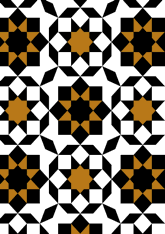data189/MOR0324

## Geometry

• The symmetry group of the tiling is *442 (p4m).
• All the internal angles of the constituent polygons are a multiple of 22.5°.
• Contains four squares.
• Contains three regular two-pointed star polygons with vertex angle of 45°.
• Contains two regular 8-pointed star polygons with vertex angle of 90°.
• There are two non-regular reflective tiles (including one kite).
• The tiling satisfies the two-colour condition.
• The tiling is edge-to-edge.
• As drawn, contains about 355 polygons.

## References

Publications referenced: